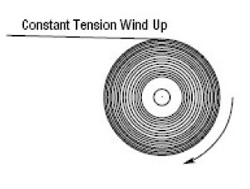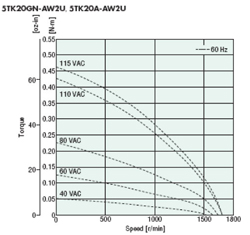Free Shipping for Online Orders. Terms and conditions apply.

# Using a Torque Motor for Winding and Unwinding Type Applications

A torque motor is an excellent product for winding and unwinding type applications.  Due to the design of the motor, it reaches full torque at 0 output speed, or locked rotor condition.  A torque motor can act as a brake when back driven, or a simple device to provide tension.  Depending on the torque setting, the motor will provide tension to the material while taking up slack in the line automatically, essentially matching the line speed.

The TMP-1 power controller can be used to adjust the torque setting by changing the voltage going to the motor.  It can be set via onboard potentiometers, external potentiometers, and external DC voltage.  The user can simply set the torque to the required level and the motor will automatically match the line speed due to its natural characteristics.

When referring to the torque-speed chart below, you will see that higher voltages will increase the motor torque and lower voltages will decrease the torque.  As the torque decreases, the effective motor speed will naturally decrease as well.  The torque level should be adjusted so that the generated torque will not break the material being wound.

This way, any slack in the system is automatically taken up and tensioned properly.  If the motor is run at 60 VAC or less (half the rated voltage), the torque motor carries a continuous duty cycle rating.

## EXAMPLEApplication Specs:

Max Diameter = 12”
Min Diameter = 4”
Max force per material = 32 oz Force
Speed = 4” / sec

Tmin = F * (D / 2)
Tmin = 64 oz-in

Tmax = F * (D / 2)
Tmax = 192 oz-in

Vmin = (4” / sec) * (rev / {4” * pi}) * 60
Vmin = 19 RPM

Vmax = (4” / sec) * (rev/ {12” * pi}) *60
Vmax = 6.4 RPM

Explanation:

If gear ratio = 15:1
Tm = (Tmin/max) / (15) / (81%) (gear efficiency)
V = (Vmin/max) * (15)

Then motor torque {Tm} and speed {V} will be:Tmin = 5.3 oz-in at 285 RPM
Tmax = 15.8 at 96 RPM

Consult the torque speed chart above. The results appear to fall within the specification of the motor when looking at the 60VAC or below curves.

0.125 Nm = 17.7 oz-in
0.05 Nm = 7.1 oz-in

Product Solution:

TMP-1 / 5TK20GN-AW2U / 5GN15SA